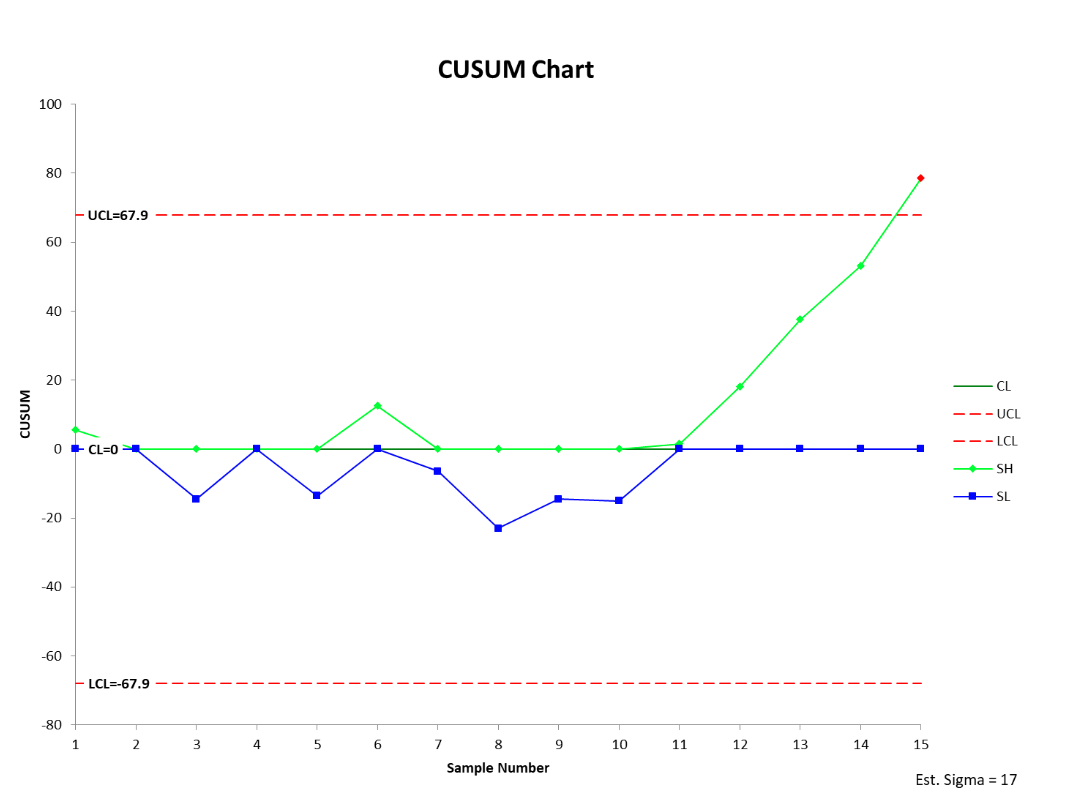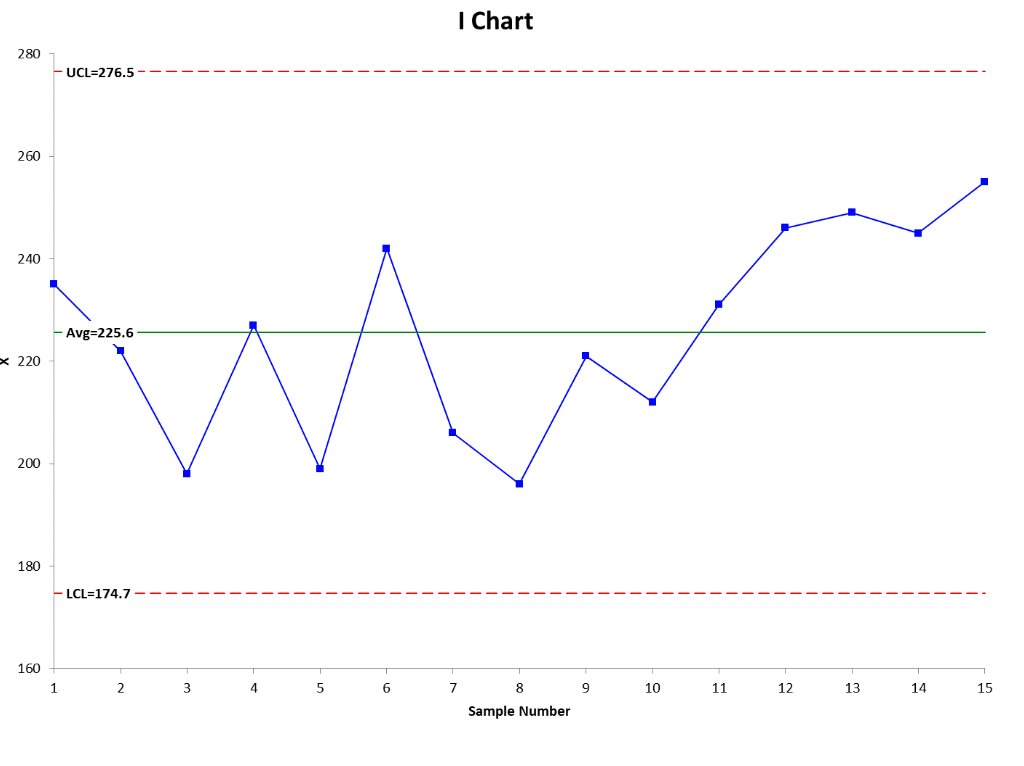Classical Shewhart charts are not very sensitive to small shifts in your process. This means you might miss a shift due to special cause variation. Small shifts are better identified by the use of a CUSUM control chart.

## Overview: What is a CUSUM chart?

A CUSUM (cumulative sum) chart is a type of control chart used to monitor the deviation from a target value. The basic advantage of a CUSUM chart is that it is more sensitive to a small shift in the process mean than traditional Shewhart charts like the I-MR or X-bar charts.

The CUSUM chart and the exponentially weighted moving average (EWMA) chart both monitor the mean of the process. The basic difference is, unlike the I-MR and X-bar charts, they consider and weigh the value of previous data at each point.

Instead of calculating the subgroup means independently, the CUSUM chart uses the values of current and previous sample data. That is why the CUSUM chart is better than the X-bar and I-MR charts for detecting small shifts in the process mean. The CUSUM chart is most effective when using individual data rather than subgroups. Some advantages of the CUSUM chart are:

• The CUSUM chart can detect small shifts in the process mean in the range of  0.5 to 2 standard deviations from the target mean
• It is easy to visually identify the shifts

Some disadvantages of the CUSUM chart are:

• Establishing and maintaining CUSUM charts is more difficult
• CUSUM charts are slower in detecting large shifts in the process mean
• Since CUSUMs are correlated, it is tough to interpret the patterns.

## An industry example of a CUSUM chart

The Master Black Belt of a large consumer products company was asked to do a control chart on some manufacturing data. He sensed the current data was more reflective of the process than prior data. He chose to do a CUSUM chart knowing it would be better at detecting any small shifts in the process than the I or X chart. Shown below are both charts. Notice how the CUSUM chart detected and signaled the process shift faster than the I chart.### What does a CUSUM chart show?

CUSUM charts identify small shifts (between .5 and 2 standard deviations) in your process means by charting a statistic that incorporates current and previous data values from the process. Specifically, it charts the cumulative sums of the deviations of the sample values from a target value.

### Which is better for detecting a small process shift – a CUSUM chart or an X-bar chart?

The CUSUM chart is better for picking up a small shift in your process but is less effective than an X-bar chart for detecting larger shifts.

### Do calculations for a CUSUM chart weight all the data the same?

No. It places more value on current data and less on prior data. That is why it is more effective in picking up smaller changes in the process.